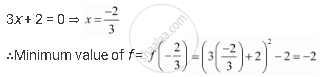Share

# Find the Maximum and Minimum Values, If Any, of the Following Functions Given by F(X) = 9x2 + 12x + 2 - CBSE (Science) Class 12 - Mathematics

#### Question

Find the maximum and minimum values, if any, of the following functions given by

f(x) = 9x2 + 12x + 2

#### Solution

The given function is f(x) = 9x2 + 12x + 2 = (3x + 2)2 − 2.

It can be observed that (3x + 2)2 ≥ 0 for every x ∈ R.

Therefore, f(x) = (3x + 2)2 − 2 ≥ −2 for every x ∈ R.

The minimum value of f is attained when 3x + 2 = 0.Hence, function f does not have a maximum value.

Is there an error in this question or solution?

#### APPEARS IN

NCERT Solution for Mathematics Textbook for Class 12 (2018 to Current)
Chapter 6: Application of Derivatives
Q: 1.2 | Page no. 231

#### Video TutorialsVIEW ALL 

Solution Find the Maximum and Minimum Values, If Any, of the Following Functions Given by F(X) = 9x2 + 12x + 2 Concept: Maxima and Minima.
S